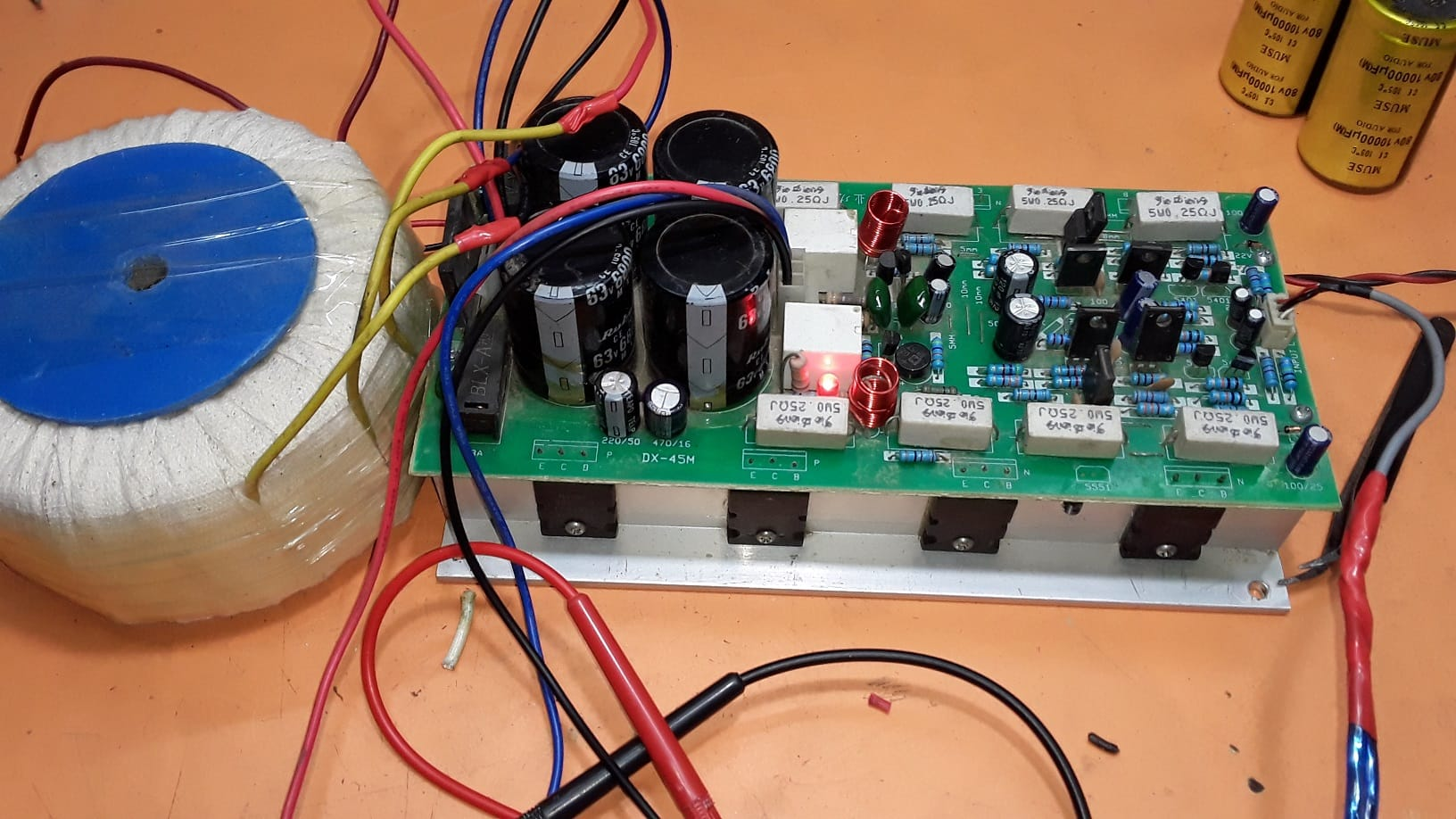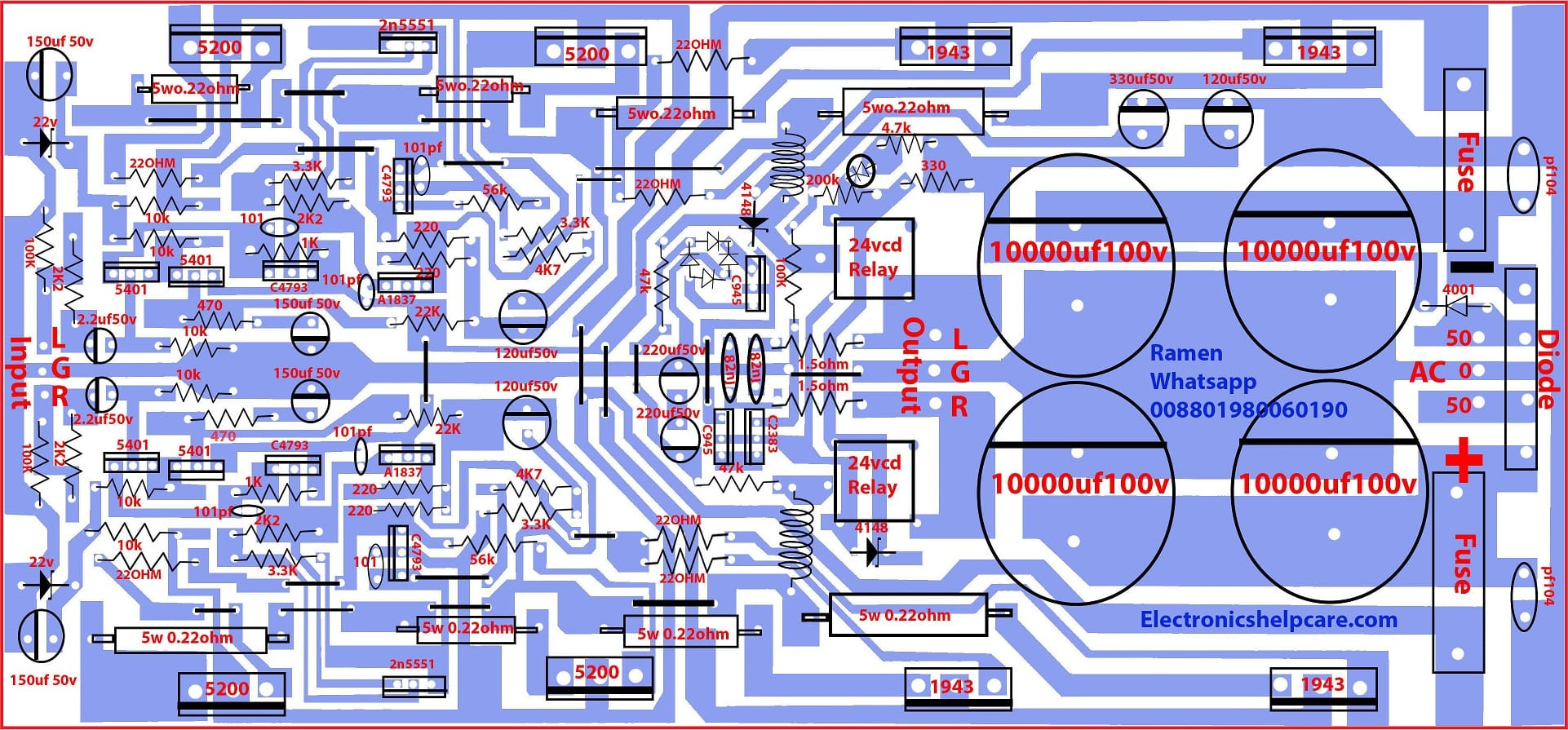Breaking News

# transistor amplifier circuit diagram

## Transistor amplifier circuit diagram:

This is a transistor amplifier. it’s a 2sc5200 and 2sa1943 transistor amplifier circuit diagram. 2sc5200 is an NPN transistor and 2sa1943 is a PNP transistor. Its Collector-Base Voltage is 230. collector amperes is 15 and base ampere is 1.5. this transistor can make a maximum of 100 Watts. this transistor’s 1st leg is the Base 2nd leg is the collector and the 3rd leg is the emitter.In this circuit, we can use 40-0-40 voltage and 5 amperes. we can’t use high voltage and amperes because the driver section transistor is so small to take high amperes. if we want to use 80-0-80 voltage, we have to use high and low voltage like 80-40-0-40-80 voltage.  This is the stereo amplifier circuit diagram. one side has 4 transistors. this circuit has 8 transistors so we can use 8 X 1.5=12.This is the circuit diagram. Here used 4 capacitors. we have to use a good quality capacitor. to make a good voltage. if we want to get a good watt then we have to use a good capacitor.We have lot of circuit diagrams. like transistor amplifier circuitSTK amplifier,  motor controllerInverter circuit diagram, Subwoofer circuit diagram,

## led music level indicator circuit diagram

This circuit needs dual voltage. positive negative and ground voltage. for this, we need a full-wave transformer to make.  Always need a good quality transformer to get a good watt. because we know that volt X ampere= Watts. here we use 40 volts 5 +5=10 amperes. So, 40X10=400 Watts.